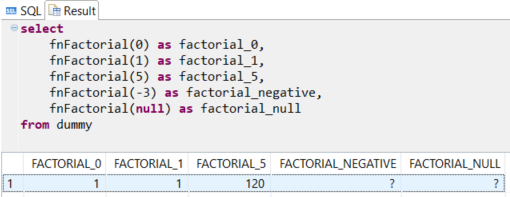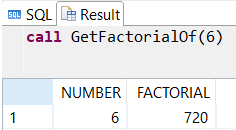SAP ABAP Programming and HANA Database Tutorials Development resources, articles, tutorials, code samples and tools and downloads for ASP.Net, SQL Server, R Script, Windows, Windows Phone, AWS, SAP HANA and ABAP, like SAP UI5, Screen Personas, etc.
 Home Articles News IT Jobs Tools Sample Chapters Trainers Blogs Forums Photos Files

Install SAP Free

# SAP HANA Database User-Defined SQL Factorial Function

In this SAP HANA database SQLScript tutorial, I want to share source codes of HANA factorial function. Using SQLScript, SAP HANA database developers can create user-defined scalar functions in order to use in inline expressions and make frequent calculations or tasks in SQL by using these HANA functions. Though table valued functions return tabular data, scalar function return single value in different HANA database data types.

As the definition of mathematical factorial function is taking a positive integer value and returning the multiplication of all integers from 1 to input parameter value, my SAP HANA factorial function accepts an integer parameter as input.

The output of the HANA factorial function is also integer.If SQLScript developers review following code, they will see an IF clause checking whether the input integer value is bigger than or equal to 0.
If the input value is zero, output is 1. From maths the value of 0! equals to 1.

Within the IF clause, a WHILE loop takes place to calculate the factorial of the given input integer parameter.

``` CREATE FUNCTION fnFactorial (  n Int ) returns factorial Int AS BEGIN if n >= 0 then  factorial := 1;  while n > 0 do   factorial := factorial * n;   n := n - 1;  end while; end if; END ```

To test our new SQL factorial function on a SAP HANA database, developers can execute following SQL query

``` select  fnFactorial(0) as factorial_0,  fnFactorial(1) as factorial_1,  fnFactorial(5) as factorial_5,  fnFactorial(-3) as factorial_negative,  fnFactorial(null) as factorial_null from dummy ```

The results are as expected as seen in below screenshotI hope this HANA database SQLScript function example will help you quickly solve your development requirements and is a good example of IF clause and WHILE clause usage within UDF functions on HANA database.

Besides above given SQLScript WHILE Loop, HANA database developers can use SERIES_GENERATE_INTEGER function with dynamic execution command EXECUTE IMMEDIATE as follows:

``` do begin declare p_i int; declare v_sql varchar(200); p_i := 6; select  'select ' || s1.ELEMENT_NUMBER || ' as Number, ' || string_agg(s2.ELEMENT_NUMBER,'*') || ' as Factorial from dummy;' into v_sql from SERIES_GENERATE_INTEGER(1, 0, 100) s1  , SERIES_GENERATE_INTEGER(1, 0, 100) s2 where s1.ELEMENT_NUMBER >= s2.ELEMENT_NUMBER  and s1.element_number = :p_i group by s1.ELEMENT_NUMBER; EXECUTE IMMEDIATE v_sql; end; ```

HANA database SQLScript programmers can wrap above SQL code block into a stored procedure as follows:

``` create procedure GetFactorialOf (  p_i int ) as begin declare v_sql varchar(200); select  'select ' || s1.ELEMENT_NUMBER || ' as Number, ' || string_agg(s2.ELEMENT_NUMBER,'*') || ' as Factorial from dummy;' into v_sql from SERIES_GENERATE_INTEGER(1, 0, 100) s1  , SERIES_GENERATE_INTEGER(1, 0, 100) s2 where s1.ELEMENT_NUMBER >= s2.ELEMENT_NUMBER  and s1.element_number = :p_i group by s1.ELEMENT_NUMBER; EXECUTE IMMEDIATE v_sql; end; ```

To execute above SQL procedure following CALL procedure command can be used as a sample

``` Call GetFactorialOf(6); ```I tried to return the result of factorial calculation into an integer variable that is defined as an output parameter of the GetFactorialOf procedure, but there is a limitation related with INTO clause in SELECT statements of "EXECUTE IMMEDIATE"

SAP DBTech JDBC: : INTO clause not allowed for this SELECT statement

So I preferred to return factorial information as a result set

Unfortunately, there are some limitations preventing to convert above SQL code into HANA database scalar function. One of the errors that you might experience with use of dynamic SQL code in a scalar function is seen below:

SAP DBTech JDBC: : feature not supported: Dynamic SQL is/are not supported in scalar function

SAP Tutorials

SAP Tutorial

SAP Forums

SAP Tools

SAP Transaction Codes Table

Meetup Sunumu 1